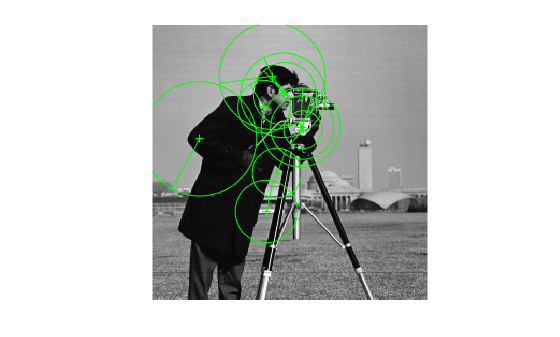# isempty

Determine if points object is empty

## Syntax

``isempty(points)``

## Description

example

````isempty(points)` returns a `true` value, if the points object is empty.```

## Examples

collapse all

`I = imread('cameraman.tif');`

Detect KAZE points from the image.

`points = detectKAZEFeatures(I);`

Check for points.

```if isempty(points) return; end```

Extract KAZE features from the detected points.

`[features,validPoints] = extractFeatures(I,points);`

Plot the ten strongest valid extracted points and show their orientations.

```imshow(I) hold on strongestPoints = selectStrongest(validPoints,10); plot(strongestPoints,'showOrientation',true) hold off```## Input Arguments

collapse all

Points object, specified as a `points` object. The object contains information about the feature points detected in the input image. To obtain points, use the appropriate detect function.

## Version History

Introduced in R2017b